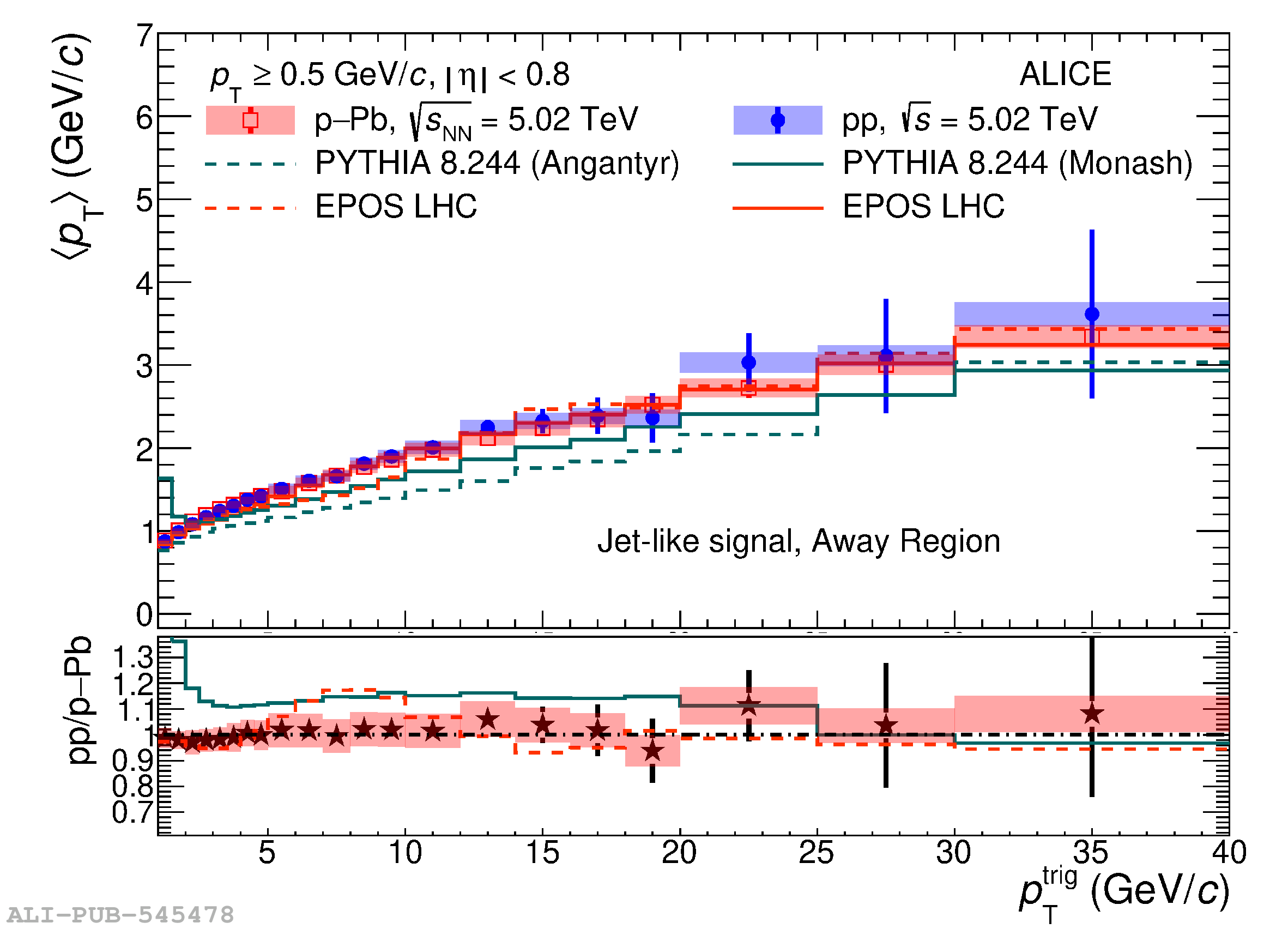# Figure 7

 Upper: average transverse momentum as a function of \ptt in the toward (left) and away (right) regions measured in \pp and \pPb collisions at $\sqrt{s_{\rm NN}}=5.02$\,\TeV. Results for data and comparison with models \py (green) and \ep (red) predictions are shown. Bottom: average transverse momentum measured in \pp collisions divided by that measured in \pPb collisions. A similar ratio is shown for model predictions.